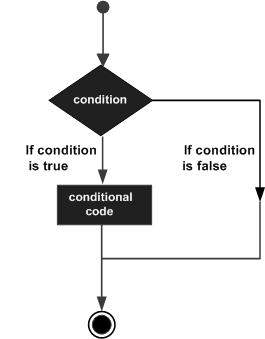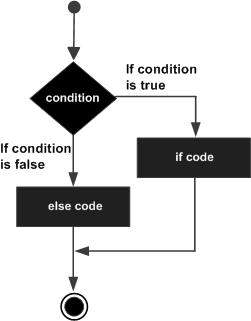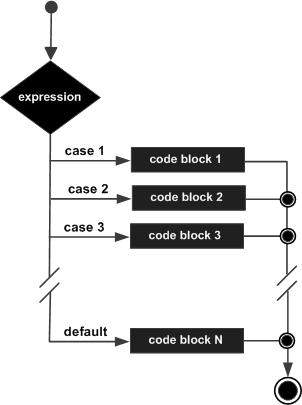# Decision Statements

Decision making is critical to computer programming. There will be many situations when you will be given two or more options and you will have to select an option based on the given conditions. For example, we want to print a remark about a student based on his secured marks. Following is the situation −
`Assume given marks are x for a student:If given marks are more than 95, thenStudent is brilliantIf given marks are less than 30, thenStudent is poorIf given marks are less than 95 and more than 30, thenStudent is average`
Now, the question is how to write a programming code to handle such situations. Almost all the programming languages provide conditional statements that work based on the following flow diagram −Let's write a C program with the help of if conditional statements to convert the above given situation into a programming code −
` `
`#include <stdio.h>int main() {   int x = 45;      if( x > 95) {       printf( "Student is brilliant\n");   }   if( x < 30) {       printf( "Student is poor\n");   }   if( x < 95 && x > 30 ) {       printf( "Student is average\n");   }}`
When the above program is executed, it produces the following result −
`Student is average`
The above program uses if conditional statements. Here, the first if statement checks whether the given condition i.e., variable x is greater than 95 or not and if it finds the condition is true, then the conditional body is entered to execute the given statements. Here we have only one printf() statement to print a remark about the student.
Similarly, the second if statement works. Finally, the third if statement is executed, here we have the following two conditions −
• First condition is x > 95
• Second condition is x < 30
The computer evaluates both the given conditions and then, the overall result is combined with the help of the binary operator &&. If the final result is true, then the conditional statement will be executed, otherwise no statement will be executed.
This tutorial will give you a basic idea on various forms of if statements and an introduction to switch statements available in C programming language. Different programming languages provide different types of decision-making statements, but the basic concept remains the same as explained in this tutorial.

## if...else statement

An if statement can be followed by an optional else statement, which executes when the Boolean expression is false. The syntax of an if...else statement in C programming language is −
`if(boolean_expression) {      /* Statement(s) will execute if the boolean expression is true */} else {    /* Statement(s) will execute if the boolean expression is false */}`
The above syntax can be represented in the form of a flow diagram as shown below −An if...else statement is useful when we have to take a decision out of two options. For example, if a student secures more marks than 95, then the student is brilliant, otherwise no such situation can be coded, as follows −
` `
`#include <stdio.h>int main() {   int x = 45;      if( x > 95) {       printf( "Student is brilliant\n");   } else {      printf( "Student is not brilliant\n");   }}`
When the above program is executed, it produces the following result −
`Student is not brilliant`

## if...elseif...else statement

An if statement can be followed by an optional else if...else statement, which is very useful to test various conditions.
While using if, else if, else statements, there are a few points to keep in mind −
• An if can have zero or one else's and it must come after an else if.
• An if can have zero to many else…if's and they must come before the else.
• Once an else…if succeeds, none of the remaining else…if's or else's will be tested.
The syntax of an if...else if...else statement in C programming language is −
`if(boolean_expression 1) {   /* Executes when the boolean expression 1 is true */}else if( boolean_expression 2) {   /* Executes when the boolean expression 2 is true */}else if( boolean_expression 3) {   /* Executes when the boolean expression 3 is true */} else {      /* Executes when the none of the above condition is true */}`
Now with the help of if...elseif...else statement, the very first program can be coded as follows −
` `
`#include <stdio.h>int main() {   int x = 45;      if( x > 95) {      printf( "Student is brilliant\n");   }    else if( x < 30) {      printf( "Student is poor\n");   }    else if( x < 95 && x > 30 ) {      printf( "Student is average\n");   }}`
When the above program is executed, it produces the following result −
`Student is average`

## The Switch Statement

A switch statement is an alternative of if statements which allows a variable to be tested for equality against a list of values. Each value is called a case, and the variable being switched on is checked for each switch case. It has the following syntax −
`switch(expression){   case ONE :      statement(s);      break;   case TWO:      statement(s);      break;   ......      default :      statement(s);}`
The expression used in a switch statement must give an integer value, which will be compared for equality with different cases given. Wherever an expression value matches with a case value, the body of that case will be executed and finally, the switch will be terminated using a break statement. If no break statements are provided, then the computer continues executing other statements available below to the matched case. If none of the cases matches, then the default case body is executed.
The above syntax can be represented in the form of a flow diagram as shown below −Now, let's consider another example where we want to write the equivalent English word for a given number. Then, it can be coded as follows −
` `
`#include <stdio.h>int main() {   int x = 2;      switch( x ){      case 1 :         printf( "One\n");         break;      case 2 :         printf( "Two\n");         break;      case 3 :         printf( "Three\n");         break;      case 4 :         printf( "Four\n");         break;      default :         printf( "None of the above...\n");   }}`
When the above program is executed, it produces the following result −
`Two`

## Decisions in Java

Following is the equivalent program written in Java which too supports if, if...else, if...elseif...else, and switch statements.
You can try to execute the following program to see the output, which must be identical to the result generated by the above C example.
` `
`public class DemoJava {   public static void main(String []args) {      int x = 45;         if( x > 95) {         System.out.println( "Student is brilliant");      }       else if( x < 30) {         System.out.println( "Student is poor");      }       else if( x < 95 && x > 30 ) {         System.out.println( "Student is average");      }   }}`
When the above program is executed, it produces the following result −
`Student is average`

## Decisions in Python

Following is the equivalent program written in Python. Python provides if, if...else, if...elif...else, and switch statements. Here, you must note that Python does not make use of curly braces for conditional body, instead it simply identifies the body of the block using indentation of the statements.
You can try to execute the following program to see the output −
` `
`x = 45if x > 95:   print "Student is brilliant"elif x < 30:   print "Student is poor"elif x < 95 and x > 30:   print "Student is average"print "The end"`
When the above program is executed, it produces the following result −
`Student is averageThe end`

`"computer systems analyst""computer systems a programmer's perspective""computer systems institute""computer systems engineering""computer systems analyst salary""computer systems analyst jobs""computer systems technician""computer systems technology""computer systems science and engineering""delta computer systems""types of computer systems""future generation computer systems""harris computer systems""integrated computer systems inc""tate computer systems""unison computer systems inc""patni computer systems""examples of computer systems""london computer systems""computer information systems""computer operating systems""computer and information systems managers""computer information systems jobs""computer information systems salary""computer science and business systems""computer information systems degree""computer science and information systems""computer information systems vs computer science""computer networks a systems approach"`